# Martin Orr's Blog

## The matrix lemma for elliptic curves

Posted by Martin Orr on Friday, 25 May 2012 at 14:00

Let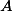be a principally polarised abelian variety of dimensionover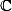. We can associate withacomplex matrix called the period matrix which roughly speaking describes a basis for the image of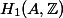in(actually it is not really the period matrix as it is only defined up to the action ofon the Siegel upper half space; we can make it nearly unique by forcing it to be in a particular fundamental domain).

The matrix lemma says that, ifis defined over a number field, then the entries of the imaginary part of the period matrix cannot be too large with respect to the height of(Faltings height or modular height).

Matrix lemma. (Masser 1987) Letbe a principally polarised abelian variety of dimensionover a number field. Letbe the period matrix forin the standard fundamental domain of the Siegel upper half space. There is a constantdepending only onsuch that all the entries ofsatisfy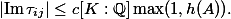Last time I used a lower bound for the lengths of non-zero periods in the proof of the isogeny theorem. This follows from the matrix lemma as we can easily relate lengths of periods and the period matrix.

Today I will prove the matrix lemma for elliptic curves. The general proof requires various facts about Siegel modular forms and also uses a funny choice of level structure due to Igusa (I do not understand why). But the basic structure of the proof is already visible in the elliptic curves case and we can be concrete about the modular forms involved, using only facts I learned in Part III.

The lemma is valid takingto be either the stable Faltings height ofor the Weil height of a point representingin the moduli space of ppAVs of dimension. This is because Faltings proved a bound for the difference between the two heights. The proof of the matrix lemma uses the height from the moduli space. But in applying it to the isogeny theorem, we need the Faltings height because we need the boundfor varieties related by an isogeny, and so far as I know this can only be proven using the Faltings height.

I was a bit disappointed when I realised this because I had thought that the Masser-Wüstholz isogeny theorem gave a proof of Finiteness Theorem I independent of Faltings' work. Of course the comparison between heights is not all of Faltings' proof, but according to Milne, "Technically, this is by far the hardest part of the proof."

In order to define the height of a point in the moduli space we need to choose an embedding of the moduli space into projective space. I think we have to choose the right embedding for the lemma to work (and also for the comparison with the Faltings height to work) but I am not very clear about that -- I think this might be related to the Igusa level structure which I mentioned.

For today, I am sticking to elliptic curves, where there is nothing to worry about. We can simply use the Weil height of the-invariant.

### The proof

Letbe an elliptic curve over the number field. Letbe a complex number in the upper half plane such thatgenerate the period lattice for. We can choosein the standard fundamental domaindefined byThe period lemma asserts that there is an absolute constantsuch that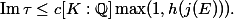We will prove this by boundingin two ways, one involvingand the other involving.

Recall that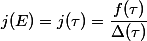whereandare modular forms of weight 12,being a certain multiple of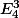andbeing a cusp form.

In the compactified fundamental domain,vanishes only at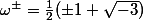. We have; indeedhas no zeroes except at the cusp. Sois non-zero in some neighbourhoods ofand. Hence there is a constantsuch thatCusp forms decay exponentially as we approach the cusp i.e. there are constants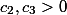such that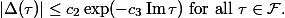(This follows from the existence of-expansions.)

Combining these two inequalities, we get that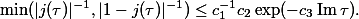Sinceis defined over the number field, the same is true of. Using the definition of the Weil height, it is not hard to prove Liouville's inequality:By basic properties of heights,so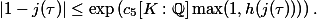HenceCombining the two bounds for, we get that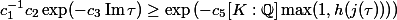which simplifies to the desired